## Year 5 maths worksheet: multiplication and division hops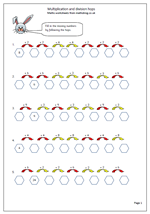Each of the questions on this page has two sets of coloured arrows above the spaces which need to be filled in. The arrows give the instruction, red for multiplication and yellow for division. There are eight hexagons, one of which has been filled in. The aim is to complete the other seven using the arrows to work out what to put inside each hexagon.

Sounds complicated but it is easier to complete than to explain!

This is good practice for

a. knowing tables

b. knowing division facts

c. knowing that multiplication and division are the inverse of each other.

This page can be found in the Year 5 Know Number Facts category.

Multiplication and division hops

## Long multiplication: 3-digits by 2-digitsA standard page of long multiplication questions here. For children to be successful with these they need to have a good knowledge of ‘times tables’. Without this knowledge the whole process becomes quite tedious and errors can slip in even if the method is correct.

The standard method of long multiplication is one which most adults will be familiar with. It does not really matter if the number is multiplied by the tens first or the units first, as long as it is remembered to place a zero in the units column when multiplying by the tens digit. It is also important to line up the answer so that hundreds, tens and units are directly under each other: adding the final total can be very difficult if this is not done.

Long multiplication 3 digits by 2 digits

## Year 2 Measurement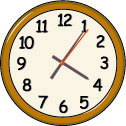Great progress is made in year 2 with measuring. Much of the work still concentrates on comparisons between different lengths, heights, weights and capacity but standard units of measurement (such as centimetres and metres) are also introduced.
Units of time are also introduced, including seconds, minutes, hours and days and the relationship between them. Reading clock faces to the quarter hour is another step forward.
I have a selection of resources on these topics, found in the Year 2 maths Worksheet category, under Measuring.
I would also especially  recommend the material at urbrainy.com for these topics, as they have a great range of resources which can be viewed free of charge and up to ten sets of worksheets printed free.

Go to Year 2 measuring resources

## Resource of the Week: Writing larger numbers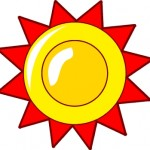Writing large numbers in words is not easy but by the end of year 5 many children will be confident enough to read numbers in the millions.

In the past, in the UK, a billion has been considered as a million million, but in the USA a thousand million has been thought of as a billion.

This seems to be the norm in the UK these days as well so Neptune at 4 500 000 000 km from the sun can be said as “four billion, five hundred million km “.

Some children become fascinated by larger numbers and like to count on up to trillions and beyond, even to the googol which is a 1 with one hundred zeros!

(10,­000,­000,­000,­000,­000,­000,­000,­000,­000,­000,­000,­000,­000,­000,­000,­000,­000,­000,­000,­000,­000,­000,­000,­000,­000,­000,­000,­000,­000,­000,­000,­000,­000)

Free Y5 maths worksheet: writing larger numbers

## Year 3 mental arithmetic: sets 15 and 16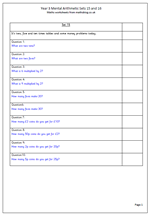Here we continue with our sets of mental arithmetic questions, suitable for Year 3 children.

This week the questions concentrate on the two, five and ten times tables as well as some money questions. The money questions look at how many coins are needed to make totals. Expect quite a fast response to these questions as tables should be know off by heart.

There are several ways for children to respond to these questions. One way is to give them a sets of number cards so that they can hold up their answer. it makes a change from writing the answers down. Teachers find this an effective way of making sure all children in the class make a response, not just leaving it to those who like to answer out loud. It can also lead to good discussions on how the answers were worked out.

Year 3 Mental arithmetic: sets 15 and 16.

## Maths SAT Paper A 2010: Questions 5 and 6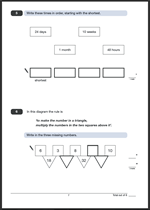Here are the latest questions and answers taken from the Maths SAT Paper A 2010.

Question 5 is a straightforward time question, putting four lengths of time in order, starting with the shortest. Look for the shortest length of time and put that down first. It is a good idea to cross that off so that it makes it easier to find the next shortest time.

Question 6 is a harder question but is worth two marks if answered correctly. Unfortunately one correct answer out of the three will not gain any marks. The first triangle number is easy to find by multiplying 3 by 8. The number in the square can be found by dividing 32 by 8.

Questions 5 and 6 Maths Paper A 2010

Questions 5 and 6 Paper A answers

## Year 3 mental arithmetic: Sets 13 and 14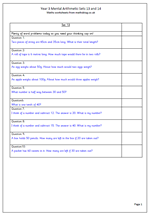Two more sets of mental arithmetic questions suitable for Year 3 children. There are plenty of word problems this week which some children find much harder than just being given a sum to work out.

Perhaps one of the hardest types of question is the’ finding the number half way between’. Another tricky type of question is the ‘I think of a number and subtract’ type. This is difficult because finding the answer does not necessarily involve carrying out the operation spoken in the question. For example, I think of a number and subtract 12. The answer is 20. The answer can be found by adding (not subtracting) 20 and 12. Watch for the common error of answering 8 for this question.

Year 3 mental arithmetic sets 13 and 14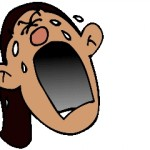A study of maths achievement in the UK, led by Carol Vorderman, has a number of interesting points to make.

Firstly it claims that  there is a systematic failure in the subject, with 300 000 children leaving school at 16 unable to function efficiently with maths in their personal lives or at work. Many pupils leave school unable to complete even the most basic sums needed for everyday life, such as adding up the change in their pocket and calculating household bills.

Secondly, we are around two years behind children in the  Far East and are less likely to study maths at a higher level than in most other developed countries.

Thirdly she suggests that all students  should study a form of maths up to the age of 18.

Finally and this is a point that I have been arguing for many years, she suggests a new functional maths for 14-16 year olds who struggle with maths. Rather than take the old GCSE they should study maths which will be useful to them in the real world, such as percentages and fractions.

Other recommendations include:

1. Rebranding maths as a “subject of critical importance” to ensure it is given higher status to other disciplines.
2. Encouraging the use of maths in other primary school subjects to ensure adding, subtracting and dividing becomes part of a “daily routine” for all children from the age of five.
3. Offering parents crash courses in the subject at school amid claims too many adults have a “fear of mathematics”.
4. Creating new syllabuses spanning the last year of primary school and start of secondary education to overcome the dip in performance between the two key stages.

## Year 5 Maths Worksheet: More In Out Function Machines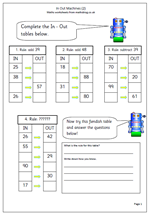I have had several requests from people for another page of In Out tables, so here it is. Each table has a set rule. A number goes in, the rule is applied and a number comes out. Straightforward if you are given the number that goes in, but a little harder if you are given the number that comes out. In that case a reverse calculation has to be done.

Some children get into a muddle with these and it is a good idea to point out that if the rule is to add then the OUT number will always be larger than the IN number. If the rule is subtract, then the OUT number will always be smaller than the IN number.

This and lots more worksheets can be found in the Year 5 Calculating category.

In out function machines (2)

## Year 1 money worksheetsChildren in Year 1 need plenty of practice with counting coins and adding up totals. There are several worksheets in the Year 1 calculating section which are ideal for this.

One shows mixed amounts of coins yo to 10p pieces to count.

The second looks at how much change should be given from 10p when spending small amounts of money.

The third is a more general mini-investigation whicjh looks at how many different ways an 8p yoyo can be bought using a selection of 1p and 2p coins.

Go to Year 1 calculating money worksheets